# The 7 Times Table

The 7 Times TableThe 7 times table is:

• 1 × 7 = 7
• 2 × 7 = 14.
• 3 × 7 = 21
• 4 × 7 = 28
• 5 × 7 = 35
• 6 × 7 = 42
• 7 × 7 = 49
• 8 × 7 = 56
• 9 × 7 = 63
• 10 × 7 = 70
• 11 × 7 = 77
• 12 × 7 = 84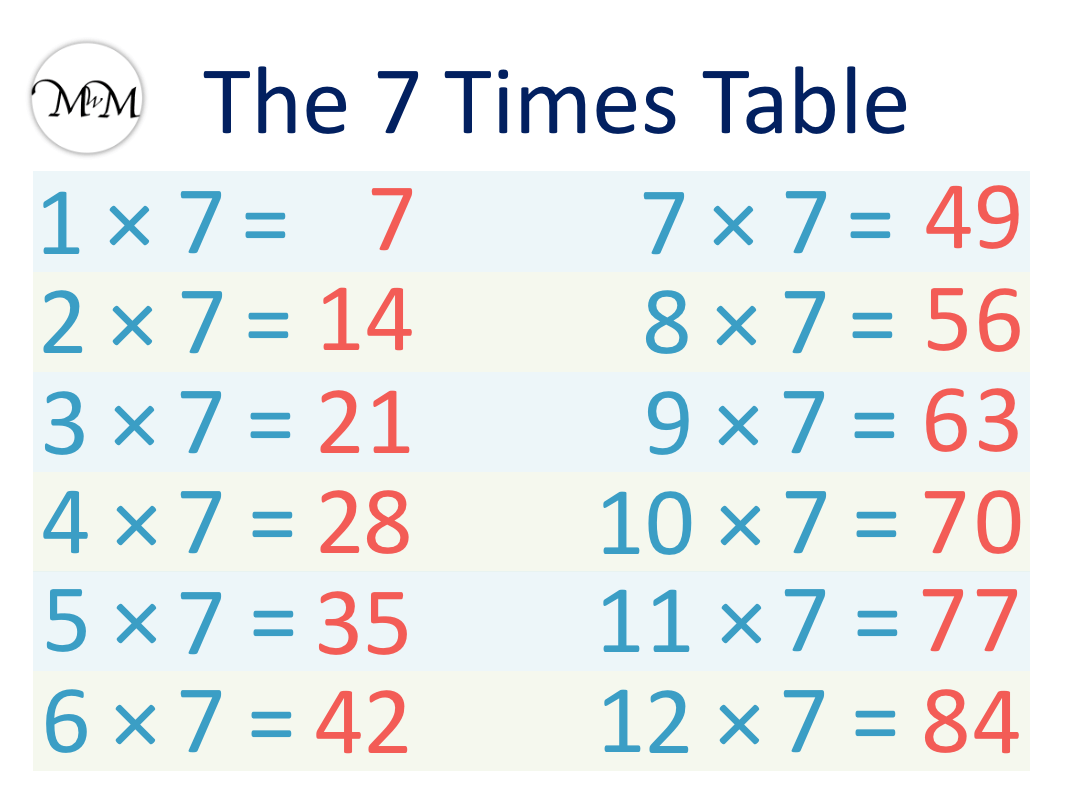• The seven times table is made by counting up in sevens.
• Start by memorising 1 × 7, 10 × 7 and 11 × 7 as these are the easiest.
• 2 × 7 is simply 7 + 7, which equals 14.
• We can then double this answer to find 4 × 7 = 28.
• When multiplying 7 by 3, 6, 9 or 12, the answers add to 3, 6, 9 or 12 respectively.
• We can remember that 8 × 7 = 56, because this contains the digits 5, 6, 7 and 8.
• We can remember 5 × 7 = 35 because it is the only one that ends in a 5.
• Finally, 7 × 7 = 49 can be found by starting with 6 × 7 = 42 and then adding 7 more.

The 7 times table is made by counting up in sevens.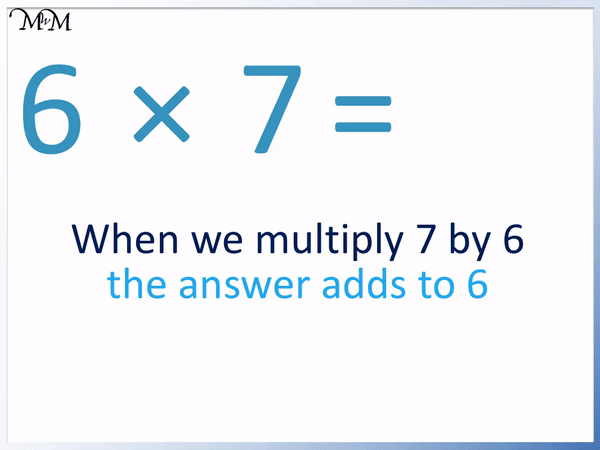• We can remember the multiples of 3 answers for 3, 6, 9 and 12 times 7.
• The digits of these answers add up to the number we are multiplying by 7.
• The answer to 6 × 7 will have digits that add up to 6.
• 6 × 7 = 42 and 4 + 2 = 6.
• This trick helps us to remember the 3, 6, 9 and 12 lots of 7.#### 7 Times Table Flashcards

Click on the 7 times table flashcards below to memorise the 7 times table.

×
×

=

# The 7 Times Table

## What is the 7 Times Table?

The sevens times table is made by counting up in sevens.

• 1 × 7 = 7
• 2 × 7 = 14.
• 3 × 7 = 21
• 4 × 7 = 28
• 5 × 7 = 35
• 6 × 7 = 42
• 7 × 7 = 49
• 8 × 7 = 56
• 9 × 7 = 63
• 10 × 7 = 70
• 11 × 7 = 77
• 12 × 7 = 84The 7 times table is generally considered one of the hardest times tables to memorise.

When teaching the 7 times table, it helps to have already memorised the other times tables so that they can be used to help learn this one.

It is recommended that the 7 times table is learnt last out of all of the times tables. This does not mean that the other times tables have to be perfect before introducing it however. Practising all of the times tables together can help reinforce them.

## How to Memorise the 7 Times Table

To memorise the 7 times table, start by learning 1 × 7 = 7, 10 × 7 = 70 and 11 × 7 = 77. These answers are in other times tables that are the easiest to remember. Next we can remember 2 × 7 = 14 by doubling 7 and 4 × 7 = 28 by doubling this answer again.

There is a trick to remembering 3, 6, 9 and 12 times 7, which is that the digits of these answers add up to 3, 6, 9 and 12.

Finally, learn 5, 7 and 8 times 7. We can remember 5 × 7 = 35 is the only answer that ends in a 5, 8 × 7 = 56 contains the digits 5, 6, 7 and 8. 7 × 7 = 49 can be found by adding 7 on to 6 × 7 = 42.

We will now look at some of these 7 times tables tricks in more detail.

We will go through the 7 times table in the order that is best to learn them.

Here are the simplest answers in the seven times table.

1 × 7 = 7 because multiplying a number by 1 does not change the number.Multiplying 7 by 1 just equals 7.

The next easiest multiplication to remember from the 7 times table is 10 × 7 = 70.

Remember that to multiply a digit by 10 we just put a zero on the end of the digit.We put a 0 ont he end after 7 to get 70. 10 × 7 = 70.

Multiplying a single digit by 11 is easy because we just repeat the digit.

11 × 7 = 77. We just repeat 7 so it is written twice.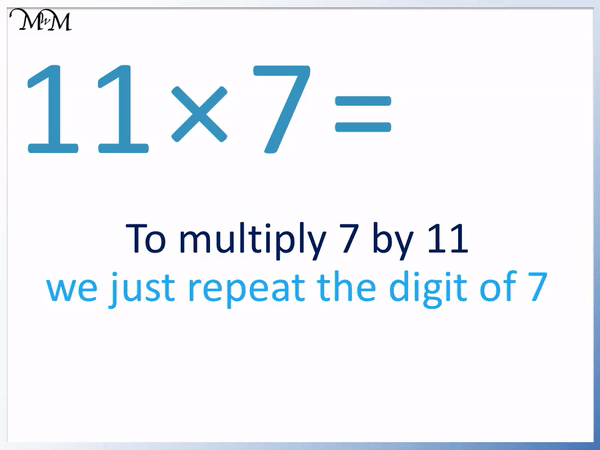After learning 1 × 7 = 7, 10 × 7 = 70 and 11 × 7 = 77, the next easiest to learn are 2 × 7 and 4 × 7.

Multiplying by 2 means to double the number. Doubling is easy because we just add the number to itself.

7 + 7 = 14 and so, 2 × 7 = 14.4 × 7 is twice as big as 2 × 7.

This means that we can find the answer to 4 × 7 by doubling the answer to 2 × 7.

14 + 14 = 28 and so, 4 × 7 = 28.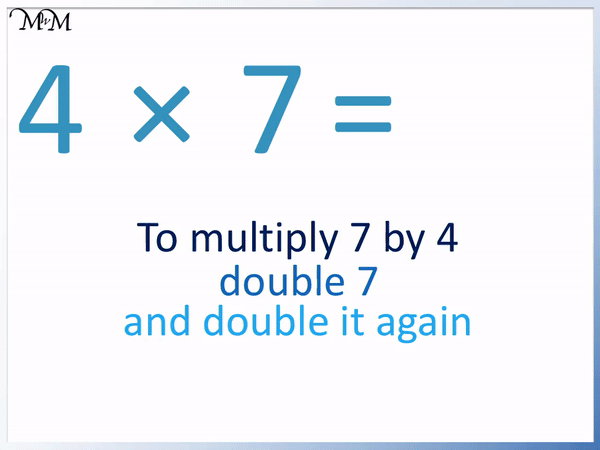The next easiest multiplications to remember in the 7 times table are the multiples of 3. This is because we have a 7 times table trick for these numbers.

We have:

3 × 7 = 21

6 × 7 = 42

9 × 7 = 63

12 × 7 = 84

We can remember these answers because the digits in each answer add to the number that we have multiplied by 7.

3 × 7 = 21 and 2 + 1 = 3

6 × 7 = 42 and 4 + 2 = 6

9 × 7 = 63 and 6 + 3 = 9

12 × 7 = 84 and 8 + 4 = 12

Here is 3 × 7 = 21.Here is 6 × 7 = 42.Here is 9 × 7 = 63.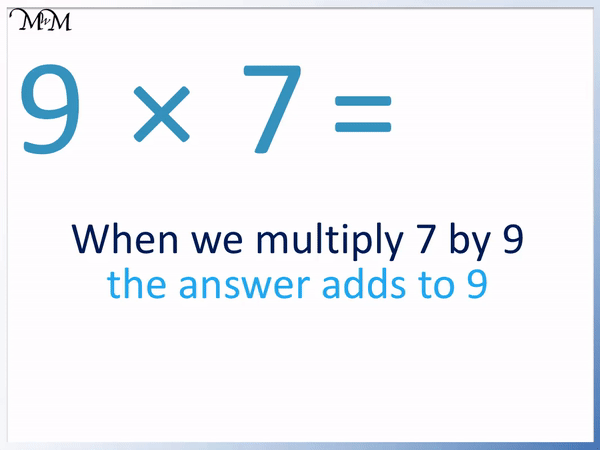Here is 12 × 7 = 84.

We can also work out 12 × 7 by multiplying 7 by 10 and by 2 and then adding the two results together.10 × 7 = 70 and 2 × 7 = 14.

70 + 14 = 84 and so, 12 × 7 = 84.

There are 3 more times tables to learn in the 7 times table.

5 × 7 = 35 is the next easiest to learn because it is the only number in the 7 times table that ends in a 5.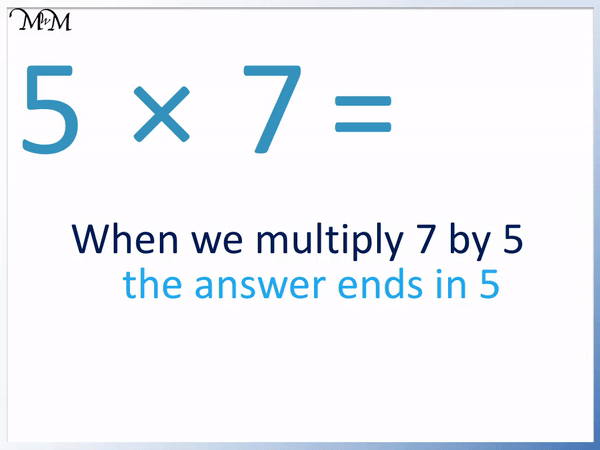We can easily multiply a number by 5 by multiplying it by 10 and halving it.

10 × 7 = 70 and half of 7 is 35.

The next easiest to remember is 8 × 7 = 56.

We can remember this because the question and the answer all contain the digits 5, 6, 7 and 8.

We just have to remember that the digits 5, 6, 7 and 8 are not in the correct order in the multiplication.Finally, the most challenging times table to remember is 7 × 7 = 49.

With the 7 times table being one of the most difficult times tables to remember, 7 × 7 = 49 is often remembered the least well out of all times tables.

The best way to work out 7 × 7 = 49 is to remember 6 × 7 = 42 and to simply add on another 7 to this.

2 + 7 = 9 and so 42 + 7 = 49.When teaching the 7 times table, it is a good idea to frequently review 7 × 7 = 49 and 8 × 7 = 56. Since there are not many quick times table tricks for these two times tables, it is best to learn these two with repetition and times table games until you are fluent.Now try our lesson on Halving Odd Numbers where we learn how to find half of an odd number.error: Content is protected !!